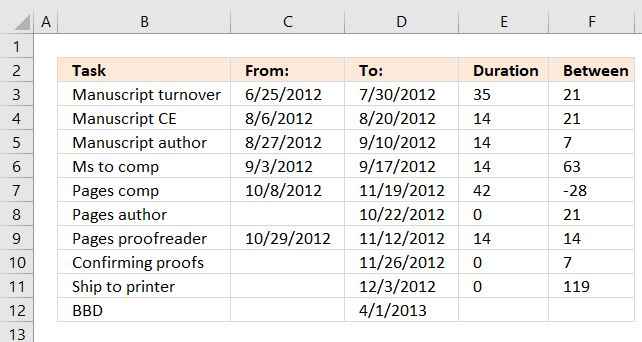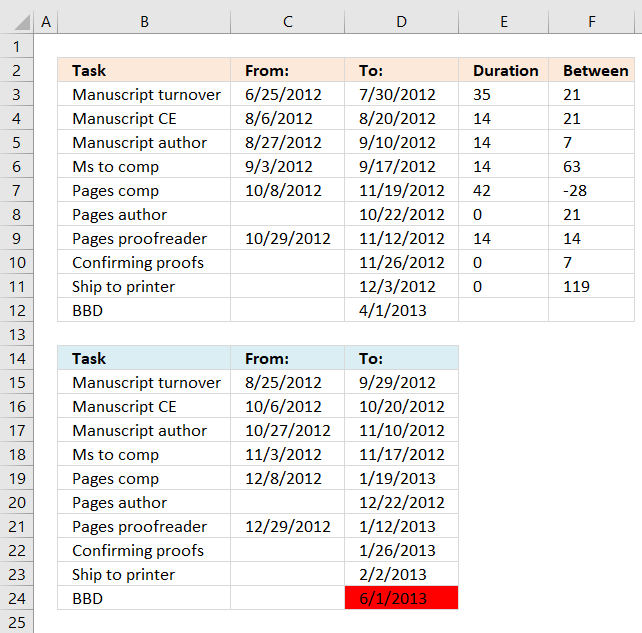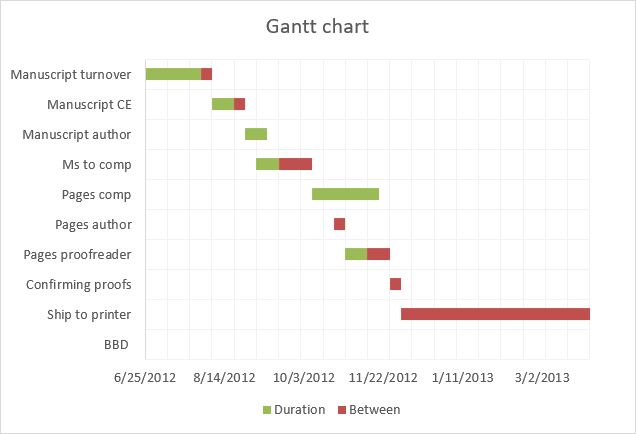Author: Oscar Cronquist Article last updated on May 07, 2019I have a schedule that I am working with and based on one date (ie. 6/4/12) different processes take different times to complete (ie. one step could only take a week, another could take up to 4 weeks).

Is there a formula I can use to calculate each step in the process based off of the date range of completion for the first step in the process?

So for example, if you look at the BBD date at the bottom, all of the steps above it take a certain amount of time to complete and have to be finished on time in order for the project to be complete by 1/4/2013.

Instead of typing in manually the date ranges I am trying to write a formula that will allow me to input the project date (ie 1/4/2013) and have all of the other steps populate themselves based on how long they take to complete (ie. the manuscript to CE step could take 2 weeks, the manuscript from CE could take 1 week and so on). I hope that makes sense??

ie. ONE ROUND From: To:
Manuscript turnover 6/25/2012 7/30/2012
Manuscript to CE 8/6/2012
Manuscript from CE 8/20/2012
Manuscript to author 8/27/2012
Manuscript from author 9/10/2012

Ms to comp 9/3/2012 9/17/2012
Pages from comp 10/8/2012
Pages from author 10/22/2012

Pages to comp 11/19/2012
Confirming proofs 11/26/2012
Ship to printer 12/3/2012

BBD 1/4/2013I calculated the duration for each step (column E) and the days between each step (column F). I then used the calculations in column E and F to calculate new dates in cell range E15:D23 based on a new finish date in cell D24.

The following formula calculates the number of days between From: and To: dates.

Formula in cell E3:

=IF(C3="",0,D3-C3)

The IF function checks if cell C3 is blank and then returns 0 (zero) if TRUE and D3-C3 if FALSE. Copy cell E3 and paste to cell range E4:E11.

The formula below calculates the number of days between tasks.

Formula in cell F3:

=D4-D3

Copy cell F3 and paste to cell range F4:F11.

Calculate new dates based on finish date

The following formula in cell C15 checks if C3 is blank and returns a blank if TRUE or returns D15-E3 if FALSE. D15-E3 calculates the From: date by subtracting the duration from the To: date.

Formula in cell C15:

=IF(C3="","",D15-E3)

Copy cell C15 and paste to cell range C16:C23.

The formula below calculates the To: date by subtracting the number of days between the tasks from the To: date of the next task.

Formula in cell D15:

=D16-F3

Copy cell D15 and paste to cell range D16:D23.

The formulas above make it possible to change the date in cell D24 and the other project dates will follow based on the calculations made in the first table.

This Gantt chart shows the processes and the days between, green days are the task duration and red days are the number of days between the current task and the next task.